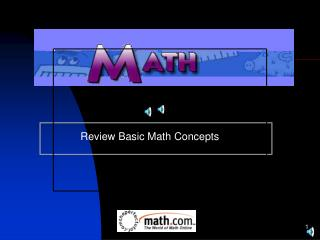Download PresentationReview Basic Math ConceptsReview Basic Math Concepts - PowerPoint PPT Presentation

Download PresentationReview Basic Math Concepts
An Image/Link below is provided (as is) to download presentation

Download Policy: Content on the Website is provided to you AS IS for your information and personal use and may not be sold / licensed / shared on other websites without getting consent from its author. While downloading, if for some reason you are not able to download a presentation, the publisher may have deleted the file from their server.

- - - - - - - - - - - - - - - - - - - - - - - - - - - E N D - - - - - - - - - - - - - - - - - - - - - - - - - - -
Presentation Transcript

1. Review Basic Math Concepts

2. Topics Covered (ONLINE RESOURCES) • Define Math • Basic Math • Rules for Addition • Addition Problems • Rules for Subtraction • Subtraction Problems • Rules for Division • Division Problems • Rules for Multiplication • Multiplication Problems

3. What is Math? Math is the science of numbers and their operations.

4. Basic Math These are basic math functions: • Addition • Subtraction • Multiplication • Division

5. What is addition? • When you add two numbers together you find how many you have in all.

6. Addition Problems • K-8 Addition • Addition Flashcards • Create your own addition problems • Online Math Game (Timed) • Baseball Game • Two Player Math Games • Counting for Kindergarteners • Math Mayhem • Basic Arithmetic Practice

7. What is Subtraction? • Subtraction is removing some objects from a group. • The meaning of 3-1=2 is “One object is taken away from a group of three objects and two objects remain".

8. Subtraction Problems • Create your own subtraction problem • Subtraction Hidden Picture • Subtracting Tens • Kids Math Level I • Subtraction Square Dance • Penguin Subtraction • Games at ToonUniversity • Math Mayhem

9. What is division? • Division means to separate something into equal parts.

10. Division Problems • Create your own division problem • Space Adventures • Math Mayhem • Division Matho

11. What is multiplication? • Multiplication is a quick way of adding identical numbers. The result of multiplying numbers is called a product.

12. Multiplication Problems • Baseball Game • Multiplication at Toon University • Lemonade Larry • Mutliplication Matho

13. Review Basic Math Concepts